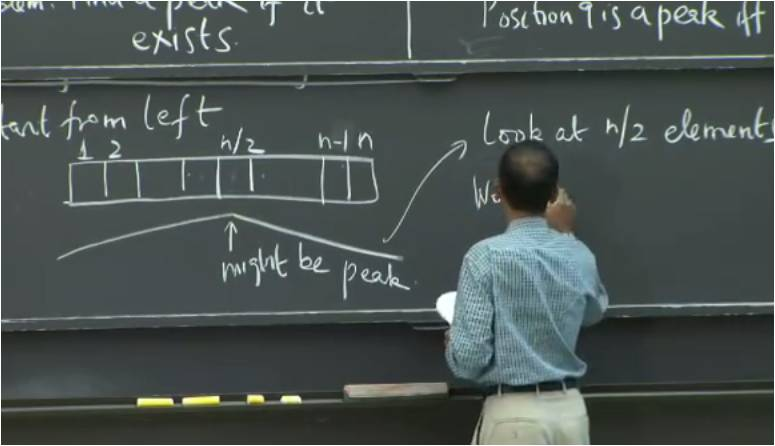# InfoCoBuild

## 6.006 Introduction to Algorithms

6.006 Introduction to Algorithms (Fall 2011, MIT OCW). Taught by Professor Erik Demaine and Professor Srinivas Devadas, this course provides an introduction to mathematical modeling of computational problems. It covers the common algorithms, algorithmic paradigms, and data structures used to solve these problems. The course emphasizes the relationship between algorithms and programming, and introduces basic performance measures and analysis techniques for these problems. (from ocw.mit.edu)Lecture 01 - Algorithmic Thinking, Peak Finding Lecture 02 - Models of Computation, Document Distance Lecture 03 - Insertion Sort, Merge Sort Lecture 04 - Heaps and Heap Sort Lecture 05 - Binary Search Trees, BST Sort Lecture 06 - AVL Trees, AVL Sort Lecture 07 - Counting Sort, Radix Sort, Lower Bounds for Sorting Lecture 08 - Hashing with Chaining Lecture 09 - Table Doubling, Karp-Rabin Lecture 10 - Open Addressing, Cryptographic Hashing Lecture 11 - Integer Arithmetic, Karatsuba Multiplication Lecture 12 - Square Roots, Newton's Method Lecture 13 - Breadth-First Search (BFS) Lecture 14 - Depth-First Search (DFS), Topological Sort Lecture 15 - Single-Source Shortest Paths Problem Lecture 16 - Dijkstra Lecture 17 - Bellman-Ford Lecture 18 - Speeding up Dijkstra Lecture 19 - Dynamic Programming I: Fibonacci, Shortest Paths Lecture 20 - Dynamic Programming II: Text Justification, Blackjack Lecture 21 - Dynamic Programming III: Parenthesization, Edit Distance, Knapsack Lecture 22 - Dynamic Programming IV: Guitar Fingering, Tetris, Super Mario Bros Lecture 23 - Computational Complexity Lecture 24 - Topics in Algorithms Research

 References Introduction to Algorithms (Fall 2011) Instructors: Prof. Erik Demaine and Prof. Srinivas Devadas. Lecture Notes. Assignments and Solutions. Exams and Solutions. Recitation Videos. Readings.